Rating : ⭐⭐⭐⭐⭐
Price : \$10.99
Language:EN
Pages: 2

# Sketch the equivalent using the notation for the transistor model appearing fig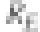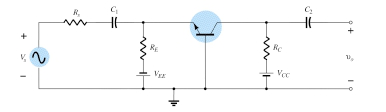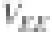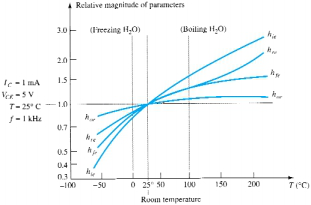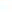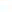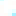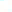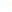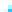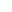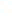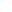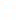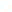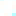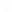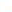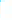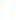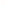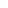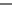Figure 7.47
§
PROBLEMS

1. (a) What is the expected amplification of a BJT transistor amplifier if the dc supply is set to zero volts?

(b) What will happen to the output ac signal if the dc level is insufficient? Sketch the effect on the waveform.

4. Given the common-base configuration of Fig. 7.48, sketch the ac equivalent using the notation for the transistor model appearing in Fig. 7.5.

Figure 7.48

Problem 4

5. (a) Describe the differences between the re and hybrid equivalent models for a BJT transistor. (b) For each model, list the conditions under which it should be applied.

§ 7.4 The Important Parameters: Zi, Zo, Av, Ai

8. Given the BJT configuration of Fig. 7.49, determine:

(a) Vi.

I = 10 i
+

BJT
transistor
amplifier

+
+ 0.6 kΩ
Vs

18 mV

Vo = 3.6 V
Vi
AυNL
Figure 7.49 Problem 8

(d) Io.

(e) Ai using the results of parts (a) and (d).
(f) Ai using Eq. (7.10).

334 iI
+ RL Io 0.51
+ 1
Zi
V s 12 mV

Vi = 4 mV

Vo
Aυ

= –180

Figure 7.50
Chapter 7
How It Works